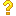•闪存
•博客
• 发言小组
• 投递新闻
• 提问博问
• 添加收藏
• 发布招聘
•文库

0

##用下面的这代码啊，画出来的是点分布图，为啥不是折线图呢？

import csv import numpy as np import pandas as pd import matplotlib.pyplot as plt import matplotlib.
1

##50Numpy dot运算在广播原则时运算并没有返回最大形状？？

1 ar1 = np.array([[1,2,3],[4,5,6],[7,8,9]]) # 形状3，3 2 ar2 = np.array([2,2,2]) #形状 1，3 3 c = np.dot(a
1

1